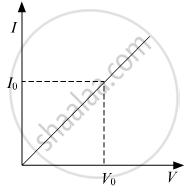# While Studying the Dependence of Potential Difference ( V) Across a Resistor on the Current (I) Passing Through It, in Order to Determine the Resistance of the Resistor, - Science

#### Question

While studying the dependence of potential difference ( V) across a resistor on the current (I) passing through it, in order to determine the resistance of the resistor, a student took 5 readings for different values of current and plotted a graph between V and t. He got a straight line graph passing through the origin. What does the straight-line signify? Write the method of determining the resistance of the resister using this graph.

#### SolutionThe straight-line graph between the potential difference ( V) and current(I) shows that resistor is a linear element and follows Ohm's Law.
Calculation of Resistance: At any point on the graph, resistance is given as,

"R" = ("V"_° ("value of potential difference at that point"))/("I"_°  ("value of current at that point"))

In other words, the Slope of the graph at any point gives the value  1/"R"
Hence,
slope ="I"_°/"V"_° = 1/"R"

⇒ "R" = 1/"slope" =( "V"_° ("Value of Potential difference at that point"))/("I"_°  ("Value of current at that point"))

Is there an error in this question or solution?